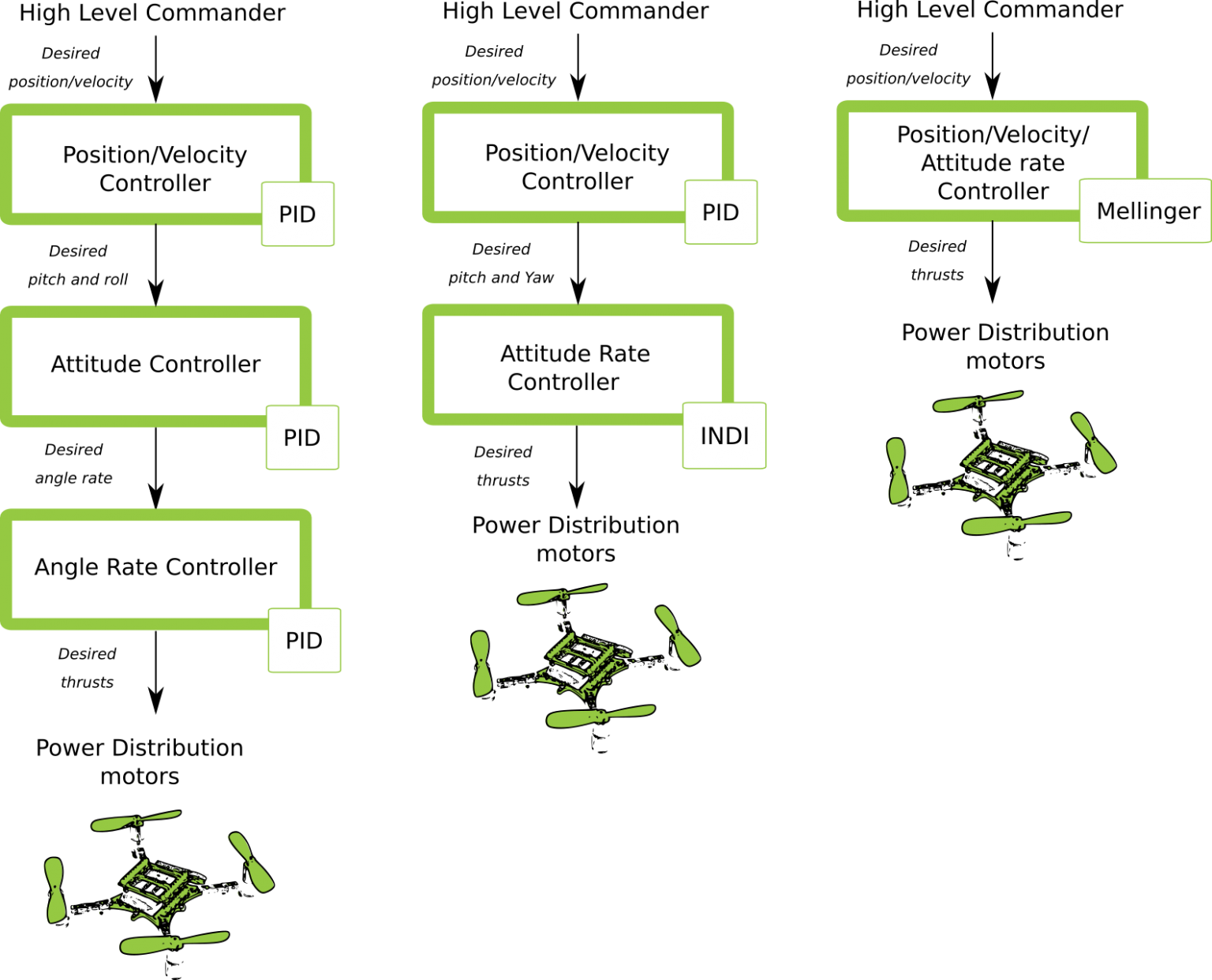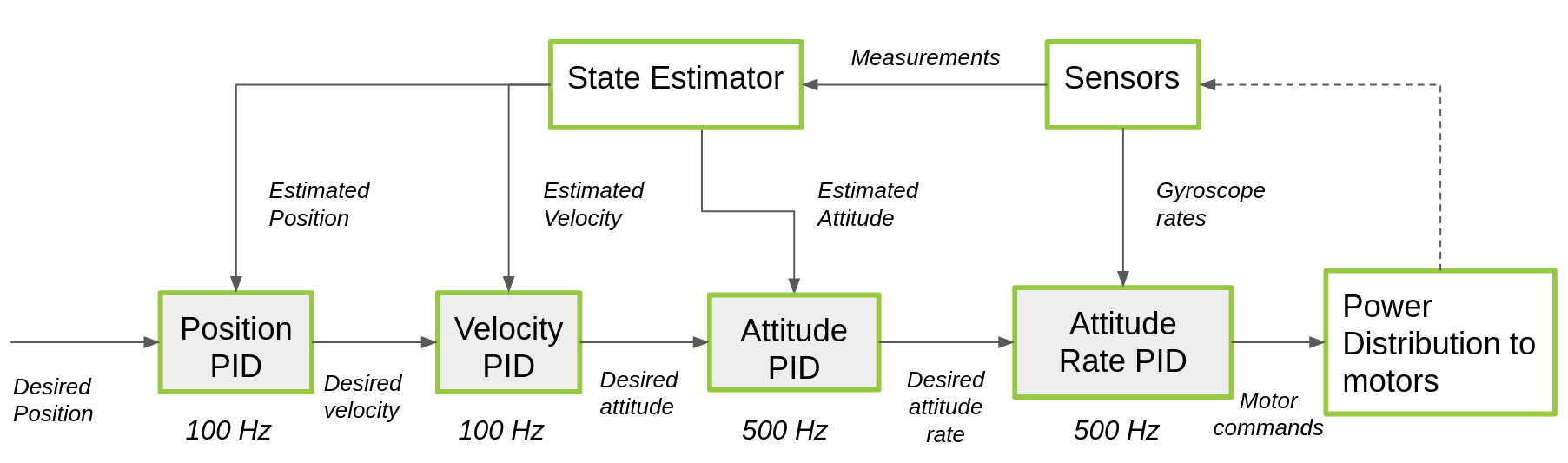# Controllers in the Crazyflie

Once the state estimator have outputed the current (estimated) situation of the crazyflie in position velocity and attitude, it is time for the controllers to keep it that way or to move the crazyflie into a new position based on a setpoint. This is an important part of the stabilization system in the crazyflie.

## Overview of control

There are four levels to control in the Crazyflie:

• Attitude rate
• Attitude absolute
• Velocity
• Position

Here is an overview of the types of controllers there are per level:We will now explain per controller how exactly they are being implemented in the crazyflie-firmware.

So the default settings in the Crazyflie firmware is the proportional integral derivative (PID) control for all desired state aspects. So the High Level Commander (HLC) or position will send desired position set-points to the PID position controller. These result in desired pitch and roll angles, which are sent directly to the attitude PID controller. These determine the desired angle rates which is send to the angle rate controller. This is also called Cascaded PID controller. That results in the desired thrusts for the roll pitch yaw and height that will be handled by the power distribution by the motors.

Here is a block schematics of how the PID controllers are implemented.Here are the different loops of the cascaded PID explained in more detail.

### Attitude Rate PID controller

The attitude rate PID controller is the one that directly controls the attitude rate. It receives almost directly the gyroscope rates (through a bit of filtering first) takes the error between the desired attitude rate as input. This output the commands that is send directly to the power distribution power_distribution_quadrotor.c. The control loop runs at 500 Hz.

Check the implementation details in attitude_pid_controller.c in attitudeControllerCorrectRatePID().

### Attitude PID controller

The absolute attitude PID controller is the outer-loop of the attitude controller. This takes in the estimated attitude of the state estimator, and takes the error of the desired attitude set-point to control the attitude of the Crazyflie. The output is desired attitude rate which is send to the attitude rate controller. The control loop runs at 500 Hz.

Check the implementation details in attitude_pid_controller.c in attitudeControllerCorrectAttitudePID().

### Position and Velocity Controller

The most outer-loop of the cascaded PID controller is the position and velocity controller. It receives position or velocity input from a commander which are handled, since it is possible to set in the variable setpoint_t which stabilization mode to use stab_mode_t (either position: modeAbs or modeVelocity). These can be found in stabilizer_types.h. The control loop runs at 100 Hz.

Check the implementation details in position_controller_pid.c in positionController() and velocityController().